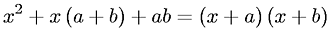Equations > Algebra > Polynomials > Second Order Polynomial Factorization

### Second Order Polynomial FactorizationLatex Code:

MathML Code:

 ${x}^{2}+x\left(a+b\right)+\mathrm{ab}=\left(x+a\right)\left(x+b\right)$

MathType 5.0: